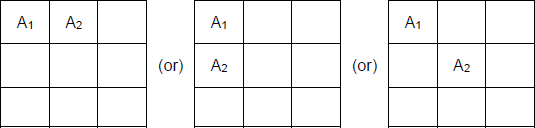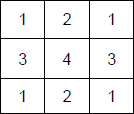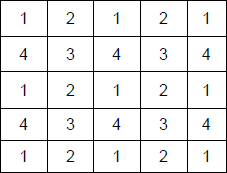### CAT 2019 - Slot 1 - Logical Reasoning and Data Interpretation - Six players – You are given an n×n square matrix

\$ 39
99
Monthly
• List Item #1
• List Item #2
Popular
##### You are given an n×n square matrix to be ?lled with numerals so that no two adjacent cells have the same numeral. Two cells are called adjacent if they touch each other horizontally, vertically or diagonally. So a cell in one of the four corners has three cells adjacent to it, and a cell in the ?rst or last row or column which is not in the corner has five cells adjacent to it. Any other cell has eight cells adjacent to it.

Q. 1: What is the minimum number of different numerals needed to ?ll a 3×3 square matrix?

##### Q. 2: What is the minimum number of different numerals needed to ?ll a 5×5 square matrix?

Q. 3: Suppose you are allowed to make one mistake, that is, one pair of adjacent cells can have the same numeral. What is the minimum number of different numerals required to ?ll a 5×5 matrix?
1. 16
2. 4
3. 25
4. 9

Q. 4: Suppose that all the cells adjacent to any particular cell must have different numerals. What is the minimum number of different numerals needed to fill a 5×5 square matrix?
1. 9
2. 16
3. 4
4. 25

Given that n × n square matrix to be filled with numerals so that no two adjacent cells have the same numeral.

Also, two cells are called adjacent if they touch each other horizontally, vertically or diagonally. As per the given definition, in the following matrix, the following are the cases of adjacent cells.Question1:

As per the information, we’ve the following diagram for a 3 x 3 matrix to have minimum number of numerals.So, we require 4 elements to have all different numerals. Ans : 4
Question 2:
As per the information, we’ve the following diagram for a 5 x 5 matrix to have minimum number of numerals.So, we require 4 elements to have all different numerals. Ans : 4

Question 3:
Even if one mistake is allowed, then also there won’t be any change in the solution given above. Ans : 4

Question 4:
Given that all the cells adjacent to any particular cell must have different numerals, which is satisfied only
when there are at least 9 numerals. Ans : 9

Inspiring Education… Assuring Success!!

Ghatkopar | Borivali | Andheri | Online